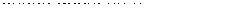# Cipher KeyA cipher key is a sequence of symbols that a user of a given cipher system must possess in order to use the system. Without a key, a user cannot encipher messages (turn them from plaintext to ciphertext) or decipher messages (turn them from ciphertext to plaintext).

Keys greatly enhance cipher security and are a feature of all modern ciphers. To see the value of keys, consider the following Caesar shift cipher:

Plaintext alphabet:
ABCDEFGHIJKLMNOPQRSTUVWXYZ

Ciphertext alphabet:
DEFGHIJKLMNOPQRSTUVWXYZABC

Note that the ciphertext alphabet is merely the plaintext alphabet shifted to the left by three letter-positions (with A, B, and C wrapped around to the right). As it stands, this cipher has no key; it consists of a one-step method that never varies (e.g., in reading the above table, E from the Plaintext alphabit always enciphers to H of the Ciphertext alphabet, the E being directly below the H in the table). Ciphers such as this example are easy to break. Twenty-four similar, but distinct ciphers can be generated, however, simply by shifting the lower alphabet by some number of positions other than three. For example, a left-shift of six letters changes the ciphertext alphabet to GHIJKLMNOPQRSTUVWXYZABCDEF. One can therefore imagine a cipher system in which one specifies a different shift before enciphering each message. The receiver will also need to know the shift, so that they can use the same substitution cipher that the sender used. In this improved cipher, the shift number for each message would function as a key . There are 25 possible keys (i.e., shifts) in this system, each of which would cause a different ciphertext to be produced from a given plaintext. This is a general feature of keys: a key modifies the rules for producing or deciphering ciphertext.

In general, an opponent who obtains a key (and who understands the rest of the cipher system) can decipher all the plaintext that has been enciphered using that key. In the example above, there are only 25 possible keys, and the cipher can easily be attacked by exhaustion, that is, by trying all possible keys. In real-world cipher systems, the number of keys is made too large for exhaustion to be practical. For example, if a 56-bit binary number is used as the key, there are 2 56 > 7.2 × 10 16 possible keys. An ideal cipher would be breakable only by exhaustion; in practice, ciphers almost always have subtle weaknesses that make it possible to break them without having to guess all possible keys.

### BOOKS:

Mollin, Richard A. An Introduction to Cryptography. New York: Chapman & Hall, 2001.